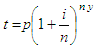# Future Value - how to calculate it using Matlab

 This program is an example of a financial application in Matlab. It calculates the future value of an investment when interest is a factor. It is necessary to provide the amount of the initial investment, the nominal interest rate, the number of compounding periods per year and the number of years of the investment.

Assuming that there are no additional deposits and no withdrawals, the future value is based on the folowing formula:where:

t = total value after y years (future value)
y = number of years
p = initial investment
i = nominal interest rate
n = number of compounding periods per year

We can achieve this task very easily with Matlab. First, we create a function to request the user to enter the data. Then, we perform the mathematical operations and deliver the result.

This is the function that requests the user to enter the necessary information:

function [ii, nir, ncppy, ny] = enter_values
ii = input(
'Enter initial investment: ');
nir = input(
'Enter nominal interest rate: ');
ncppy = input(
'Enter number of compounding periods per year: ');
ny = input(
'Enter number of years: ');

This is the function that performs the operations:

function t = future_value(ii, nir, ncppy, ny)
% Convert from percent to decimal
ni = nir / (100 * ncppy);
% Calculate future value by formula
t = ii*(1 + ni)^(ncppy * ny);
% Round off to nearest cent
t = floor(t * 100 + 0.5)/100;

And finally, this is the Matlab code (script) that handles the above:

% Instruction ‘format bank’ delivers fixed format for dollars
% and cents
% Instruction ‘format compact’ suppresses extra line-feeds

clear; clc; format compact
format bank

[ii, nir, ncppy, ny] = enter_values;
t = future_value(ii, nir, ncppy, ny)

This is a sample run:

Enter initial investment: 6800
Enter nominal interest rate: 9.5
Enter number of compounding periods per year: 4
Enter number of years: 10

with the result:

t = 17388.64

We can also test of the function, like this:

ii = 6800;
nir = 9.5;
ncppy = 4;
ny = 10;
t = future_value(ii, nir, ncppy, ny)

with exactly the same result, as expected

t = 17388.64

From 'Future Value' to home

From 'Future Value' to 'Finance Formulas'